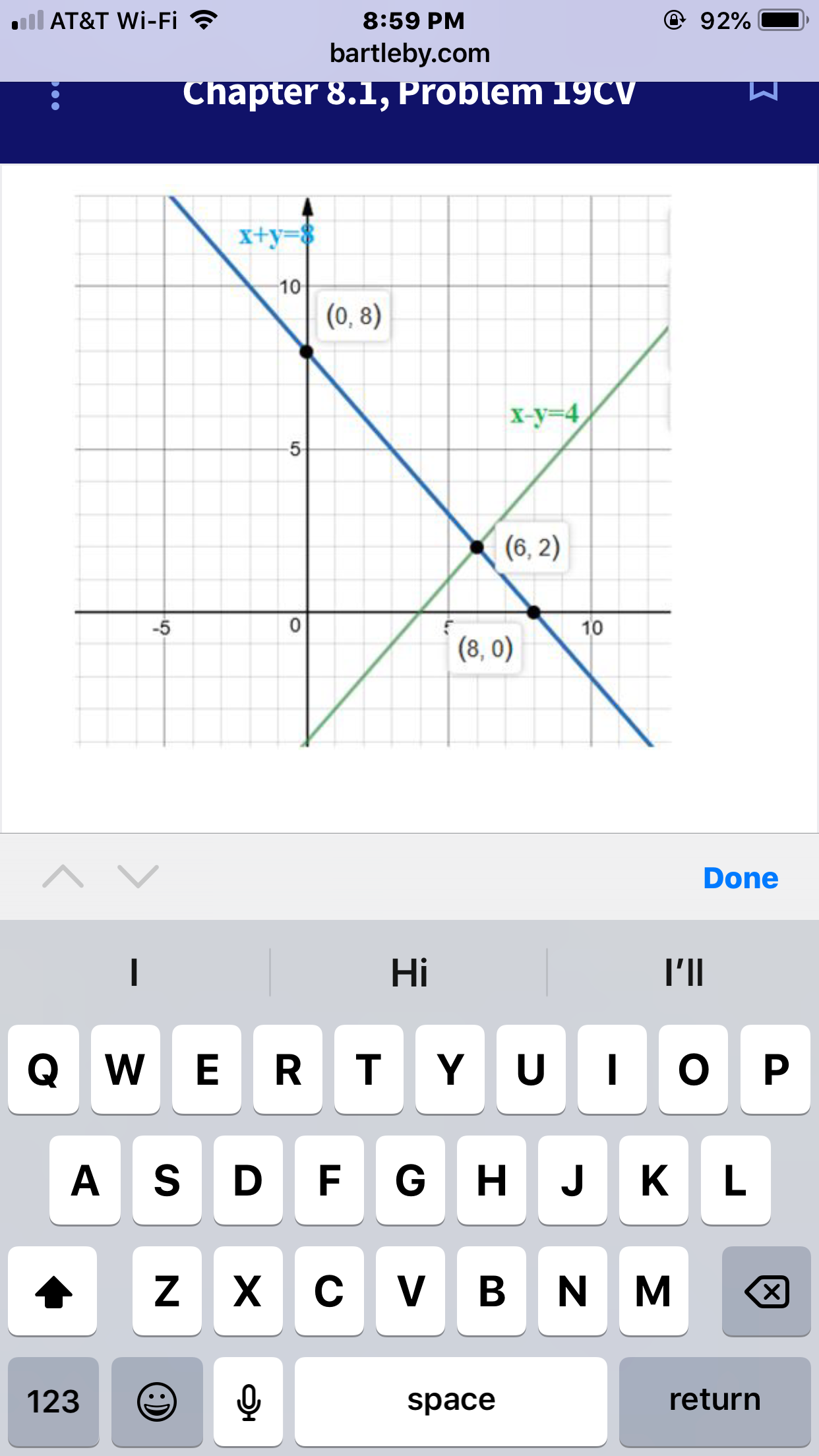# AT&T Wi-Fi8:59 PMbartleby.comhapter 8., Problem I9C10(0, 8)x-y-4(6,2)-5(8,0) 10DoneHiI'llA SDFG H J KL123returnspace

Question
32 views

Where did the other points come from

the problem is

{x+y=8

x-y=4}

i have the point (6,2) but the graph shows two other which I’m not show how to get

thankshelp_outlineImage TranscriptioncloseAT&T Wi-Fi 8:59 PM bartleby.com hapter 8., Problem I9C 10 (0, 8) x-y-4 (6,2) -5 (8,0) 10 Done Hi I'll A SDFG H J KL 123 return space fullscreen
check_circle

Step 1

It is observed that from the given graph, the ordered pairs are (0,8), (6,2) and (8,2).

Known conditions:

If the lines do not intersect, no solution exist.

If the lines intersecting at one point, unique solution exist.

If the lines are coinciding, infinite number of solutions exist.

The given graph of the lines intersecting at the ordered pair (6,2). Therefore, the solution is unique solution.

Step 2

The points (8,0) and (0, 8) are x-intercept and y-intercept of the line x + y = 8 that are not the solution of the system of equations. Also, these points not satisfied both the equations.

Step 3

Substitute x = 6 and y = 2 in both the equations to check the ordered...

### Want to see the full answer?

See Solution

#### Want to see this answer and more?

Solutions are written by subject experts who are available 24/7. Questions are typically answered within 1 hour.*

See Solution
*Response times may vary by subject and question.
Tagged in

### Other